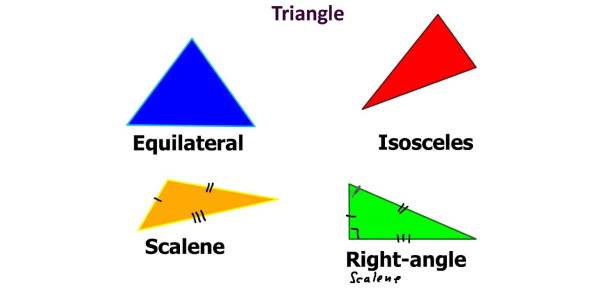Triangles Test: Can You Pass This Trivia Quiz?

15 Questions | Total Attempts: 453Settings.

• 1.
• A.
• B.
• C.
• D.
• 2.
D is a point on the side BC of a ΔABC such that AD bisects ∠BAC. Then
• A.

BD = CD

• B.

CD > CA

• C.

BD > BA

• D.

BA > BD

• 3.
It is given that Δ ABC ≅ Δ FDE and AB = 5 cm, ∠B = 40° and ∠A = 80°. Then which of the following is true?
• A.

DF = 5 cm, ∠F = 60°

• B.

DF = 5 cm, ∠E = 60°

• C.

DE = 5 cm, ∠E = 60°

• D.

DE = 5 cm, ∠D = 40°

• 4.
All the medians of a triangle are equal in case of a:
• A.

Scalene triangle

• B.

Right angled triangle

• C.

Equilateral triangle

• D.

Isosceles triangle

• 5.
In triangle PQR if ∠Q = 90o, then:
• A.

PQ is the longest side

• B.

QR is the longest side

• C.

PR is the longest side

• D.

None of these

• 6.
In the given figure, PS is the median then ∠QPS?
• A.

40o

• B.

50o

• C.

80o

• D.

90o

• 7.
In the given figure, if the exterior angle is 135o then ∠P is:
• A.

45o

• B.

60o

• C.

80o

• D.

90o

• 8.
In the given figure, AB = AC and ∠B = 50o then; ∠A is:
• A.

50o

• B.

80o

• C.

100o

• D.

130o

• 9.
In a triangle ABC, ∠B = 35o and ∠C = 60o, then
• A.

∠A = 80o

• B.

∠A = 85o

• C.

∠A = 120o

• D.

∠A = 145o

• 10.
If in ΔPQR, PQ = PR then:
• A.

∠P=∠R

• B.

∠P=∠Q

• C.

∠Q=∠R

• D.

None of these

• 11.
In triangles ABC and PQR, AB = AC, ∠C =∠P and ∠B =∠Q. The two triangles are:
• A.

Isosceles but not congruent

• B.

Isosceles and congruent

• C.

Congruent but not isosceles

• D.

Neither congruent nor isosceles

• 12.
In triangles ABC and DEF, AB = FD and ∠A =∠D. The two triangles will be congruent by SAS axiom if:
• A.

BC = EF

• B.

AC = DE

• C.

AC = EF

• D.

BC = DE

• 13.
If DE = QR, EF = PR and FD = PQ, then
• A.
• B.
• C.
• D.
• 14.
• A.

80o

• B.

40o

• C.

50o

• D.

100o

• 15.
Two sides of a triangle are of length 5 cm and 1.5 cm. The length of the third side of the triangle cannot be:
• A.

3.6 cm

• B.

4.1 cm

• C.

3.8 cm

• D.

3.4 cm

Related TopicsBack to top